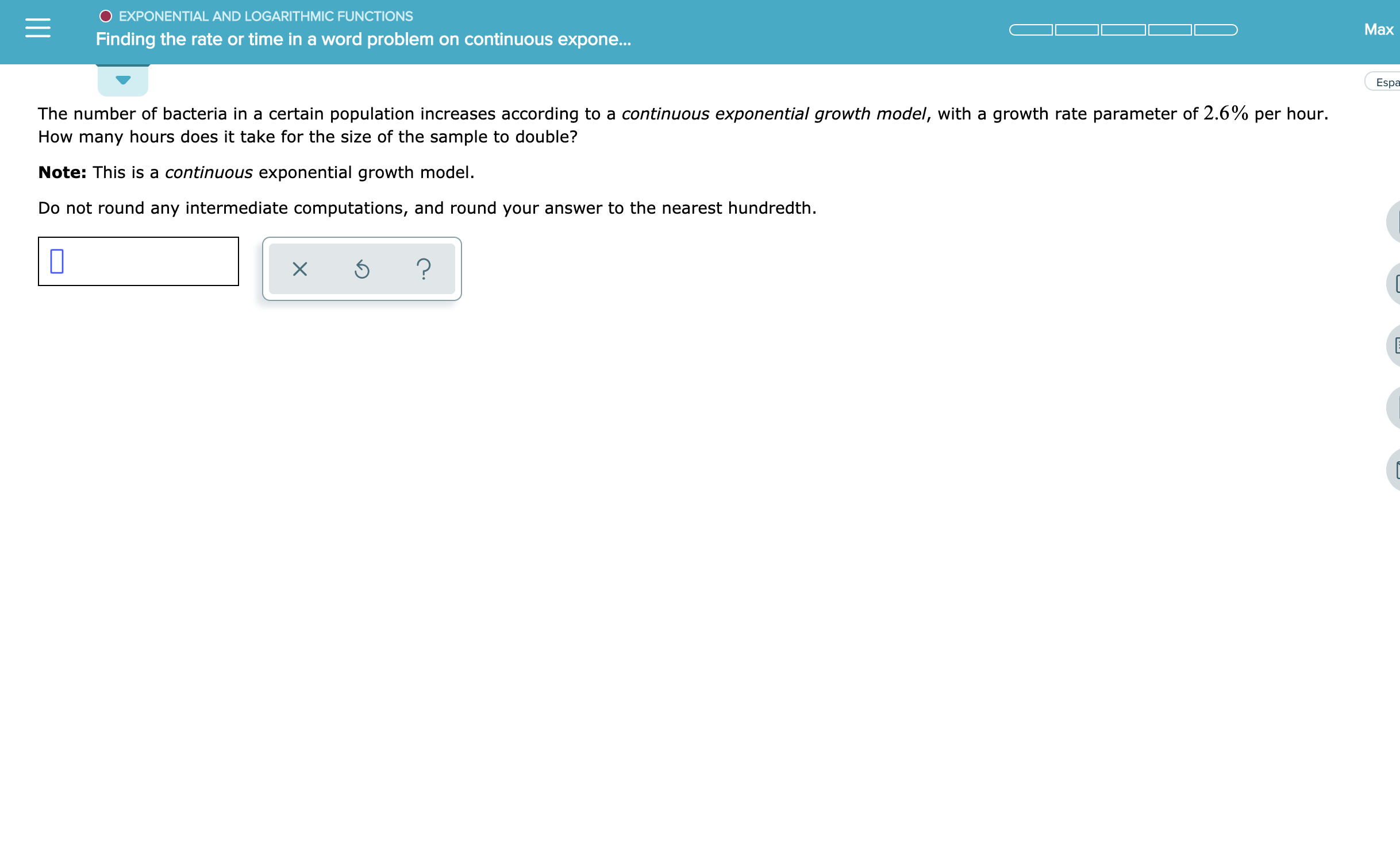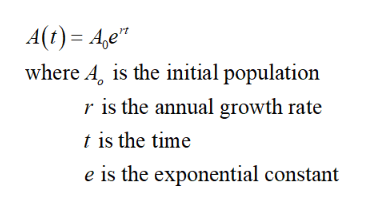# O EXPONENTIAL AND LOGARITHMIC FUNCTIONSMaxon continuous expone...Finding the rate or time in a word problemEspaThe number of bacteria in a certain population increases according to a continuous exponential growth model, with a growth rate parameter of 2.6% per hour.How many hours does it take for the size of the sample to double?Note: This is a continuous exponential growth model.Do not round any intermediate computations, and round your answer to the nearest hundredth.?

Question
9 views

see attachmenthelp_outlineImage TranscriptioncloseO EXPONENTIAL AND LOGARITHMIC FUNCTIONS Max on continuous expone... Finding the rate or time in a word problem Espa The number of bacteria in a certain population increases according to a continuous exponential growth model, with a growth rate parameter of 2.6% per hour. How many hours does it take for the size of the sample to double? Note: This is a continuous exponential growth model. Do not round any intermediate computations, and round your answer to the nearest hundredth. ? fullscreen
check_circle

Step 1

The continuous exponential gr...help_outlineImage TranscriptioncloseA(t) A,e" where A, is the initial population r is the annual growth rate t is the time e is the exponential constant fullscreen

### Want to see the full answer?

See Solution

#### Want to see this answer and more?

Solutions are written by subject experts who are available 24/7. Questions are typically answered within 1 hour.*

See Solution
*Response times may vary by subject and question.
Tagged in

### Other